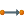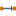You are viewing the RapidMiner Studio documentation for version 9.2 - Check here for latest version

#Data to Similarity (RapidMiner Studio Core)

## Synopsis

This operator measures the similarity of each example of the given ExampleSet with every other example of the same ExampleSet.

## Description

The Data to Similarity operator calculates the similarity among examples of an ExampleSet. Same comparisons are not repeated again e.g. if example x is compared with example y to compute similarity then example y will not be compared again with example x to compute similarity because the result will be the same. Thus if there are n examples in the ExampleSet, this operator does not return n^2 similarity comparisons. Instead it returns (n)(n-1)/2 similarity comparisons. This operator provides many different measures for similarity computation. The measure to use for calculating the similarity can be specified through the parameters. Four types of measures are provided: mixed measures, nominal measures, numerical measures and Bregman divergences.

The behavior of this operator can be considered close to a certain scenario of the Cross Distances operator, if the same ExampleSet is provided at both inputs of the Cross Distances operator and the compute similarities parameter is also set to true. In this case the Cross Distances operator behaves similar to the Data to Similarity operator. There are a few differences though e.g. in this scenario examples are also compared with themselves and secondly the signs (i.e.+ive or -ive) of the results are also different.

## Differentiation

###Data to Similarity Data

The Data to Similarity Data operator calculates the similarity among all examples of an ExampleSet. Even examples are compared to themselves. Thus if there are n examples in the ExampleSet, this operator returns n^2 similarity comparisons. The Data to Similarity Data operator returns an ExampleSet which is merely a view, so there should be no memory problems.

## Input

•example set (IOObject)

This input port expects an ExampleSet. It is the output of the Retrieve operator in the attached Example Process. The output of other operators can also be used as input.

## Output

•similarity (Similarity Measure)

A similarity measure object that contains the calculated similarity between each example of the given ExampleSet with every other example of the same ExampleSet is delivered through this port.

•example set (IOObject)

The ExampleSet that was given as input is passed without changing to the output through this port. This is usually used to reuse the same ExampleSet in further operators or to view the ExampleSet in the Results Workspace.

## Parameters

• measure_typesThis parameter is used for selecting the type of measure to be used for calculating similarity. following options are available: mixed measures, nominal measures, numerical measures and Bregman divergences. Range: selection
• mixed_measureThis parameter is available if the measure type parameter is set to 'mixed measures'. The only available option is the 'Mixed Euclidean Distance' Range: selection
• nominal_measureThis parameter is available if the measure type parameter is set to 'nominal measures'. This option cannot be applied if the input ExampleSet has numerical attributes. In this case the 'numerical measure' option should be selected. Range: selection
• numerical_measureThis parameter is available if the measure type parameter is set to 'numerical measures'. This option cannot be applied if the input ExampleSet has nominal attributes. In this case the 'nominal measure' option should be selected. Range: selection
• divergenceThis parameter is available if the measure type parameter is set to 'bregman divergences'. Range: selection
• kernel_typeThis parameter is only available if the numerical measure parameter is set to 'Kernel Euclidean Distance'. The type of the kernel function is selected through this parameter. Following kernel types are supported:
• dot: The dot kernel is defined by k(x,y)=x*y i.e.it is the inner product of x and y.
• radial: The radial kernel is defined by exp(-g ||x-y||^2) where g is the gamma that is specified by the kernel gamma parameter. The adjustable parameter gamma plays a major role in the performance of the kernel, and should be carefully tuned to the problem at hand.
• polynomial: The polynomial kernel is defined by k(x,y)=(x*y+1)^d where d is the degree of the polynomial and it is specified by the kernel degree parameter. The Polynomial kernels are well suited for problems where all the training data is normalized.
• neural: The neural kernel is defined by a two layered neural net tanh(a x*y+b) where a is alpha and b is the intercept constant. These parameters can be adjusted using the kernel a and kernel b parameters. A common value for alpha is 1/N, where N is the data dimension. Note that not all choices of a and b lead to a valid kernel function.
• sigmoid: This is the sigmoid kernel. Please note that the sigmoid kernel is not valid under some parameters.
• anova: This is the anova kernel. It has the adjustable parameters gamma and degree.
• epachnenikov: The Epanechnikov kernel is this function (3/4)(1-u2) for u between -1 and 1 and zero for u outside that range. It has the two adjustable parameters kernel sigma1 and kernel degree.
• gaussian_combination: This is the gaussian combination kernel. It has the adjustable parameters kernel sigma1, kernel sigma2 and kernel sigma3.
• multiquadric: The multiquadric kernel is defined by the square root of ||x-y||^2 + c^2. It has the adjustable parameters kernel sigma1 and kernel sigma shift.
Range: selection
• kernel_gammaThis is the SVM kernel parameter gamma. This parameter is only available when the numerical measure parameter is set to 'Kernel Euclidean Distance' and the kernel type parameter is set to radial or anova. Range: real
• kernel_sigma1This is the SVM kernel parameter sigma1. This parameter is only available when the numerical measure parameter is set to 'Kernel Euclidean Distance' and the kernel type parameter is set to epachnenikov, gaussian combination or multiquadric. Range: real
• kernel_sigma2This is the SVM kernel parameter sigma2. This parameter is only available when the numerical measure parameter is set to 'Kernel Euclidean Distance' and the kernel type parameter is set to gaussian combination. Range: real
• kernel_sigma3This is the SVM kernel parameter sigma3. This parameter is only available when the numerical measure parameter is set to 'Kernel Euclidean Distance' and the kernel type parameter is set to gaussian combination. Range: real
• kernel_shiftThis is the SVM kernel parameter shift. This parameter is only available when the numerical measure parameter is set to 'Kernel Euclidean Distance' and the kernel type parameter is set to multiquadric. Range: real
• kernel_degreeThis is the SVM kernel parameter degree. This parameter is only available when the numerical measure parameter is set to 'Kernel Euclidean Distance' and the kernel type parameter is set to polynomial, anova or epachnenikov. Range: real
• kernel_aThis is the SVM kernel parameter a. This parameter is only available when the numerical measure parameter is set to 'Kernel Euclidean Distance' and the kernel type parameter is set to neural. Range: real
• kernel_bThis is the SVM kernel parameter b. This parameter is only available when the numerical measure parameter is set to 'Kernel Euclidean Distance' and the kernel type parameter is set to neural. Range: real

## Tutorial Processes

### Introduction to the Data to Similarity operator

The 'Golf' data set is loaded using the Retrieve operator. A breakpoint is inserted here so that you can have a look the ExampleSet. You can see that the ExampleSet has 14 examples. The Data to Similarity operator is applied on it to compute the similarity of examples. As there are 14 examples in the given ExampleSet, there will be 91 (i.e. (14)(14-1)/2) similarity comparisons in the resultant similarity measure object which can be seen in the Results Workspace.